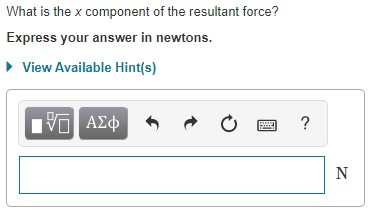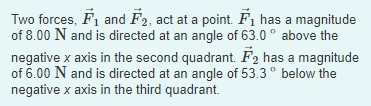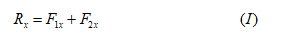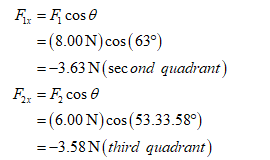# What is the x component of the resultant force?Express your answer in newtons.• View Available Hint(s) Two forces, F1 and F2, act at a point. Fi has a magnitudeof 8.00 N and is directed at an angle of 63.0 ° above thenegative x axis in the second quadrant. F2 has a magnitudeof 6.00 N and is directed at an angle of 53.3° below thenegative x axis in the third quadrant.

Question
69 views

Two forces, F⃗ 1F→1F_1_vec and F⃗ 2F→2F_2_vec act at a point. F⃗ 1F→1F_1_vec has a magnitude of 8.00 NN and is directed at an angle of 63.0 ∘∘ above the negative x-axis in the second quadrant. F⃗ 2F→2F_2_vec has a magnitude of 6.00 NN and is directed at an angle of 53.3 ∘∘ below the negative x-axis in the third quadrant.help_outlineImage TranscriptioncloseWhat is the x component of the resultant force? Express your answer in newtons. • View Available Hint(s) fullscreenhelp_outlineImage TranscriptioncloseTwo forces, F1 and F2, act at a point. Fi has a magnitude of 8.00 N and is directed at an angle of 63.0 ° above the negative x axis in the second quadrant. F2 has a magnitude of 6.00 N and is directed at an angle of 53.3° below the negative x axis in the third quadrant. fullscreen
check_circle

Step 1

The x component of the resultant force is.F1x and F2x can be calculated as follows....

### Want to see the full answer?

See Solution

#### Want to see this answer and more?

Solutions are written by subject experts who are available 24/7. Questions are typically answered within 1 hour.*

See Solution
*Response times may vary by subject and question.
Tagged in

### Physics